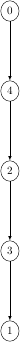Queries for Lattices: search statistic / browse statistics / browse maps from / browse maps to

# Definition & Example

• A (finite) lattice is a finite set $P$ together with a partial order $\leq$ satisfying

• $\leq$ is reflexive: $x \leq x$ for all $x \in P$,
• $\leq$ is transitive, $x \leq y \leq z$ implies $x \leq z$ for all $x,y,z \in P$,
• $\leq$ is antisymmetric, $x \leq y \Rightarrow y \not\leq x$ for $x,y \in P$ such that $x \neq y$,

such that any two elements $x$ and $y$ have a least upper bound $x \vee y$, called join, and a greatest lower bound $x \wedge y$, called meet.

• One often writes $a < b$ for $a \leq b$ and $a \neq b$.

• A cover relation $a \prec b$ is a pair of elements $a < b$ such that there exists no $c \in P$ for which $a < c < b$ [Wei14].

 the 5 Lattices of size 5([(0,1),(0,2),(0,3),(1,4),(2,4),(3,4)],5) ([(0,2),(0,3),(1,4),(2,4),(3,1)],5) ([(0,3),(1,4),(2,4),(3,1),(3,2)],5) ([(0,4),(2,3),(3,1),(4,2)],5) ([(0,2),(0,3),(2,4),(3,4),(4,1)],5)

• Lattices are graphically represented by their Hasse diagram which is the directed graph of cover relations.

• Two lattices $(P,\leq_P)$ and $(P',\leq_{P'})$ are isomorphic if there exists a bijection $\pi: P\ \tilde\longrightarrow\ P'$ such that $x \leq_P y$ if and only if $\pi(x) \leq_{P'} \pi(y)$ for all $x,y \in P$.

• This project considers unlabelled lattices. This is, two lattices are considered to be equal if they are isomorphic.

• For the number of unlabelled lattices see OEIS:A006966.

## Notations

• $x,y \in P$ are called comparable if $x \leq y$ or $y \leq x$. A poset is called linear, linearly ordered, or totally ordered if any two elements are comparable.

• [Wei14] E. Weisstein, Cover relation, Math World (2014)

# Technical information for database usage

• A lattice is uniquely represented as a tuple (E,n) where E is the sorted list of cover relations and n is the number of elements. For this representation, we consider a canonical labelling of a poset. This is, a labelling of the elements by $\{0,1,\ldots,n-1\}$ such that any two lattices are isomorphic if and only if their canonical labellings coincide.
• Lattices are graded by the number of elements.
• The database contains all posets of size at most 9.

If you want to edit this wiki page, you can download the raw markdown and send your new version to info@findstat.org